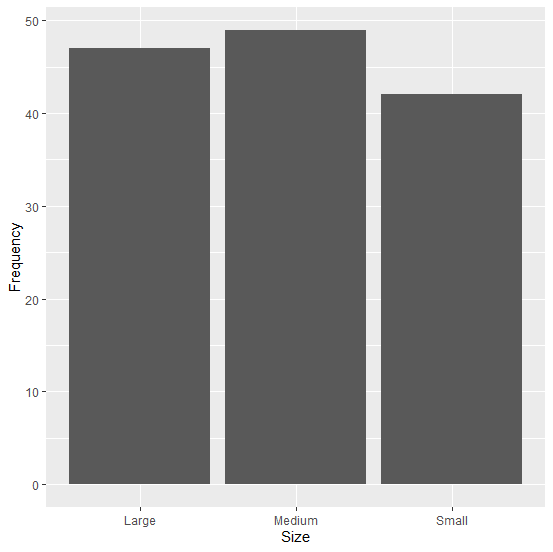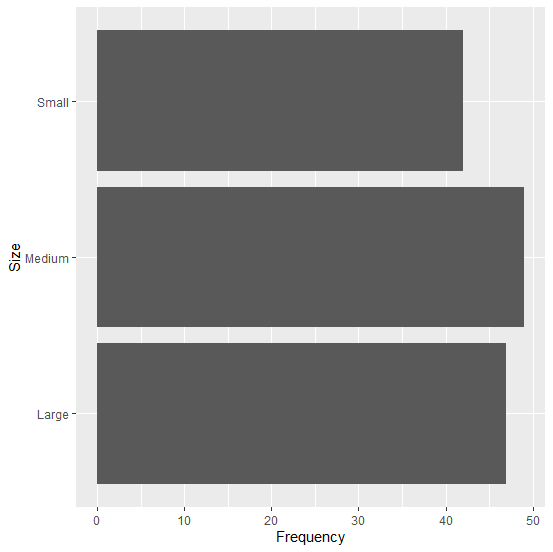# How to create a horizontal bar graph using ggplot2 in R?

Making comparisons is bit easier through horizontal bar graphs as compared to the vertical bar graphs in cases where the labels for the categories have large names. Because a large name for the labels of a vertical bar graph is likely to mix with the other labels and therefore, the reading of these labels become difficult for the viewer. To solve this problem, we can draw a bar graph and flip it with coord_flip() in ggplot2.

## Example

Consider the below data frame −

Size <-c("Small","Medium","Large")
Frequency <-c(42,49,47)
df <-data.frame(Size,Frequency)
df

## Output

Size Frequency
1 Small 42
2 Medium 49
3 Large 47
> library(ggplot2)

Creating vertical bar graph −

ggplot(df,aes(Size,Frequency))+geom_bar(stat="identity")

## OutputCreating horizontal bar graph −

ggplot(df,aes(Size,Frequency))+geom_bar(stat="identity")+coord_flip()

## Output